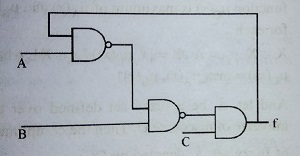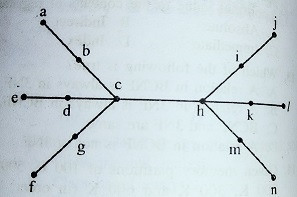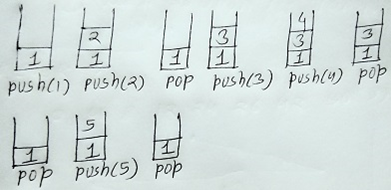### UGC NET December 2012 Paper II solved

UGC – NET
Computer Science & Applications, December 2012
PAPER-II
Q1. Consider the circuit shown below. In a certain a steady state, Y is at logical ‘l’. What are possible values of A, B, C?(A) A=0, B=0, C=1
(B) A=0, B=C, C=1
(C) A=1, B=C=0
(D) A=B=1, C=1
Ans: (A)
Q2. The worst case time complexity of AVL tree is better in comparison to binary search tree for
(A) Search and Insert Operations
(B) Search and Delete Operations
(C) Insert and Delete Operations
(D) Search, Insert and Delete Operations
Ans: (D)
Q3. The GSM network is divided into the following three major systems:
(A) SS, BSS, OSS
(B) BSS, BSC, MSC
(C) CELL, BSC, OSS
(D) SS, CELL, MSC
Ans: (A)
Q4. The power set of {ϕ} is
(A) { ϕ}
(B) {ϕ, {ϕ}}
(C) {0}
(D) {0, ϕ, {ϕ}}
Ans: (B)
Q5. If the disk head is located initially at 32, find the number of disk moves required with FCFS if the disks queue of I/O blocks requests are 98, 37, 14, 124, 65, 67.
(A) 239
(B) 310
(C) 321
(D) 325
Ans: (C)
Q6. Component level design is concerned with
(A) Flow oriented analysis
(B) Class based analysis
(C) Both of the above
(D) None of the above
Ans: (C)
Q7. The ‘C’ language is
(A) Context free language
(B) Context sensitive language
(C) Regular language
(D) None of the above
Ans: (A)
Q8. The Mobile Application Protocol (MAP) typically runs on top of which protocol?
(A) SNMP (Simple Network Management Protocol)
(B) SMTP (Simple Mail Transfer Protocol)
(C) SS7 (Signalling System 7)
(D) HTTP (Hyper Text Transfer Protocol)
Ans: (C)
Q9. If a packet arrives with an M-bit value is ‘l’ and a fragmentation offset value ‘0’, and then it is ______ fragment.
(A) First
(B) Middle
(C) Last
(D) All of the above
Ans: (A)
Q10. The number of bit strings of length eight that will either start with a 1 bit or end with two bits 00 shall be
(A) 32
(B) 64
(C) 128
(D) 160
Ans: (D)
Q11. In compiler design ‘reducing the strength’ refers to
(A) Reducing the range of values of input variables.
(B) Code optimization using cheaper machine instructions.
(C) Reducing efficiency of program.
(D) None of the above
Ans: (B)
Q12. In which addressing mode, the effective address of the operand is generated by adding a constant value to the contents of register?
(A) Absolute
(B) Indirect
(C) Immediate
(D) Index
Ans: (D)
Q13. Which of the following is true?
(A) A relation in BCNF is always in 3NF.
(B) A relation in 3NF is always in BCNF.
(C) BCNF and 3NF are same.
(D) A relation in BCNF is not in 3NF.
Ans: (A)
Q14. Given memory partitions of 100 K, 500 K, 200 K, 300 K and 600 K (in order) and processes of 212 K, 417 K, 112 K, and 426 K (in order), using the first-fit algorithm, in which partition would the process requiring 426 K be placed?
(A) 500 K
(B) 200 K
(C) 300 K
(D) None of these
Ans: (D)
Q15. What is the size of the Unicode character in Windows Operating System?
(A) 8-Bits
(B) 16-Bits
(C) 32-Bits
(D) 64-Bits
Ans: (16)
Q16. In which tree, for every node the height of its left sub tree and right sub tree differ almost by one?
(A) Binary search tree
(B) AVL tree
(D) Complete Binary Tree
Ans: (B)
Q17. The design issue of Data link Layer in OSI Reference Model is
(A) Framing
(B) Representation of bits
(C) Synchronization of bits
(D) Connection control
Ans: (A)
Q18. Given the following expressions of a grammar
E -> E * F / F + E / F
F -> F – F / id
Which of the following is true?
(A) * has higher precedence than +
(B) – has higher precedence than *
(C) + and – have same precedence
(D) + has higher precedence than *
Ans: (B)
Q19. The maturity levels used to measure a process are
(A) Initial, Repeatable, Defined, Managed, Optimized.
(B) Primary, Secondary, Defined, Managed, Optimized.
(C) Initial, Stating, Defined, Managed, Optimized.
(D) None of the above
Ans: (A)
Q20. The problem of indefinite blockage of low-priority jobs in general priority scheduling algorithm can be solved using:
(A) Parity bit
(B) Aging
(C) Compaction
(D) Timer
Ans: (B)
Q21. Which API is used to draw a circle?
(A) Circle ( )
(B) Ellipse ( )
(C) Round Rect ( )
(D) Pie ( )
Ans: (B)
Q22. In DML, RECONNCT command cannot be used with
(A) OPTIONAL Set
(B) FIXED Set
(C) MANDATOR Set
(D) All of the above
Ans: (B)
Q23. Coaxial cable are categorized by Radio Government rating are adapted for specialized functions. Category RG-59 with impedance 75 Ohm used for
(A) Cable TV
(B) Ethernet
(C) Thin Ethernet
Thick Ethernet
Ans: (A)
(A) Rapid and Design
(B) Rapid Aided Development
(C) Rapid Application Development
(D) Rapid Application Design
Ans: (C)
Q25. Suppose that someone starts with a chain letter. Each person who receives the letter is asked to send it on to 4 other people. Some people do this, while some do not send any letter.
How many people have seen the letter, including the first person, if no one receives more than one letter and if the chain letter ends after there have been 100 people who read it but did not send it out? Also find how many people sent out the letter?
(A) 122 & 22
(B) 111 & 11
(C) 133 & 33
(D) 144 & 44
Ans: (A)
Q26. A hash function f defined as f (key) = key mod 13, with linear probing is used to insert keys 55, 58, 68, 91, 27, 145. What will be the location of 79?
(A) 1
(B) 2
(C) 3
(D) None of these
Ans: (A)
Explanation: Modulus 13 of 55, i.e. f(55) = 3, f(58)=6, f(68)=3,f(91)=0,f(27)=1,f(145)=2, f(79)=1
Q27. Which of the following is true while converting CFG to LL (I) grammar?
(A) Remove left recursion alone
(B) Factoring grammar alone
(C) Both of the above
(D) None of the above
Ans: (C)
Q28. Identify the Risk factors which are associated with Electronic payment system.
(A) Fraudulent use of Credit Cards.
(B) Sending Credit Card details over internet.
(C) Remote storage of Credit Card details.
(D) All of the above
Ans: (D)
Q29. Which of the following are two special functions that are meant for handling exception that occurs during exception handling itself?
(A) Void terminate ( ) and Void unexpected ( )
(B) Non void terminate ( ) and void unexpected ( )
(C) Void terminate ( ) and non-void unexpected ( )
(D) Non void terminate ( ) and non-void unexpected ( )
Ans: (A)
Q30. Which of the following memory allocation scheme suffers from external fragmentation?
(A) Segmentation
(B) Pure demand paging
(C) Swapping
(D) Paging
Ans: (A)
Explanation: Paging suffer with internal fragmentation. For more info visit software engineering section in CBSE selected question of this website.
Q31. Basis path testing falls under
(A) System testing
(B) White box testing
(C) Black box testing
(D) Unit testing
Ans: (B)
Q32. The User Work Area (UWA) is a set of Program variables declared in the host program to communicate the contents of individual records between
(A) DBMS & the Host record
(B) Host program and Host record
(C) Host program and DBMS
(D) Host program and Host language
Ans: (B)
Q33. Consider the tree given below:Using the property of eccentricity of a vertex, find every vertex that is the center of the given tree.
(A) d & h
(B) c & k
(C) g, b, c, h, I, m
(D) c & h
Ans: (A)
Explanation: Other nodes are at same distance from c & h.
Q34. The maximum number of keys stored in a B-tree of order m and depth d is
(A) md + 1 – 1
(B) (md+1 – 1) / (m – 1)
(C) (m – 1) (md + 1 – 1)
(D) (md – 1)/(m – 1)
Ans: (All options are incorrect)
Explanation: The maximum number of keys in a B-tree can be found using formula md+1 -1.
Q35. Which of the following is the most powerful paring method?
(A) LL (I)
(B) Canonical LR
(C) SLR
(D) LALR
Ans: (B)
Q36. In UNIX, which of the following command is used to set the task priority?
(A) init
(B) nice
(C) kill
(D) PS
Ans: (D)
Q37. AES is a round cipher based on the Rijndal Algorithm that uses a 128-bit block of data. AES has three different configurations. ______ rounds with a key size of 128 bits, ______ rounds with a key size of 192 bits and ______ rounds with a key size of 256 bits.
(A) 5, 7, 15
(B) 10, 12, 14
(C) 5, 6, 7
(D) 20, 12, 14
Ans: (B)
Q38. Match the following IC families with their basic circuits:
 a. TTL 1 NAND b. ECL 2 NOR c. CMOS 3 Inverter
Code:
 a b C (A) 1 2 3 (B) 3 2 1 (C) 2 3 1 (D) 2 1 3
Ans: (C)
Q39. Match the following with respect to C++ data types:
 a. User defined type 1 Qualifier b. Built in type 2 Union c. Derived type 3 Void d. Long double 4 Pointer
Code:
 a b c D (A) 2 3 4 1 (B) 3 1 4 2 (C) 4 1 2 3 (D) 3 4 1 2
Ans: (A)
Explanation: Union is user defined, void is built in, pointer is derived and qualified is long double data types.
Q40. Given an empty stack, after performing push (1), push (2), Pop, push (3), push (4), Pop, Pop, push (5), pop, what is the value of the top of the stack?
(A) 4
(B) 3
(C) 2
(D) 1
Ans: (D)
Explanation: See the image below:Q41. Enumeration is a process of
(A) Declaring a set of numbers
(B) Sorting a list of strings
(C) Assigning a legal values possible for a variable
(D) Sequencing a list of operators
Ans: (C)
Q42. Which of the following mode declaration is used in C++ to open a file for input?
(A) ios: : app
(B) in:: ios
(C) ios: : file
(D) ios: : in
Ans: (D)
Q43. Data Encryption Techniques are particularly used for ______.
(A) Protecting data in Data Communication System.
(B) Reduce Storage Space Requirement.
(C) Enhances Data Integrity.
(D) Decreases Data Integrity.
Ans: (A)
Q44. Let L be a set accepted by a nondeterministic finite automaton. The number of states in non-deterministic finite automaton is |Q|. The maximum number of states in equivalent finite automaton that accepts L is
(A) |Q|
(B) 2|Q|
(C) 2|Q| – 1
(D) 2|Q|
Ans: (C)
Q45. What is the result of the following expression?
(1 & 2) + (3 & 4)
(A) 1
(B) 3
(C) 2
(D) 0
Ans: (A)
Q46. Back propagation is a learning technique that adjusts weights in the neural network by propagating weight changes.
(A) Forward from source to sink No
(B) Backward from sink to source from position p
(C) Forward from source to hidden nodes
(D) Backward from since to hidden nodes
Ans: (C)
Q47. Match the following:
 a. TTL 1 High fan out b. ECL 2 Low propagation delay c. CMOS 3 High power dissipation
Code:
 a b C (A) 3 2 1 (B) 1 2 3 (C) 1 3 2 (D) 3 1 2
Ans: (D)
Q48. ______ is an “umbrella” activity that is applied throughout the software engineering process.
(A) Debugging
(B) Testing
(C) Designing
(D) Software quality assurance
Ans: (D)
Q49. Identify the operation which is commutative but not associative?
(A) OR
(B) NOR
(C) EX-OR
(D) NAND
Ans: (B)
Q50. Given a Relation POSITION (Posting No, Skill), then query to retrieve all distinct pairs of posting-nos. requiring skill is
(A) Select p.posting-No, p.posting-No From position p where p.skill= p.posting-No
(B) Select p1-posting-No, p2.posting-No from position p1, position p2 where p1.skill=p2.skill
(C) Select p1.posting-No, p2.posting-No from position p1, position p2 where p1.skill=p2.skill and p1.posting-No< p2.posting-No
(D) Select p1.posting-No, p2.posting-No from position p1, position p2 where p1.skill=p2.skill and p1.posting-No=p2.posting-No
Ans: (D)